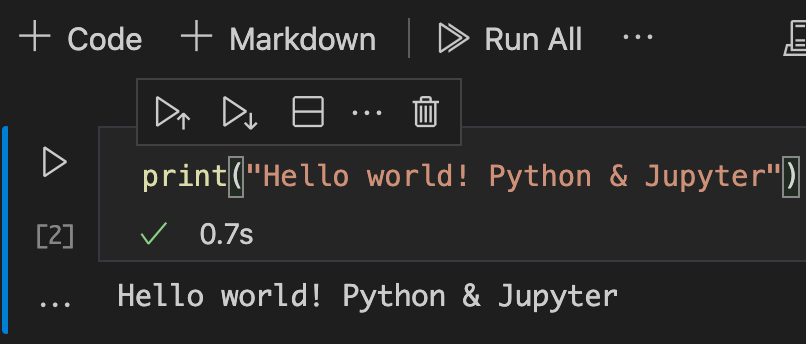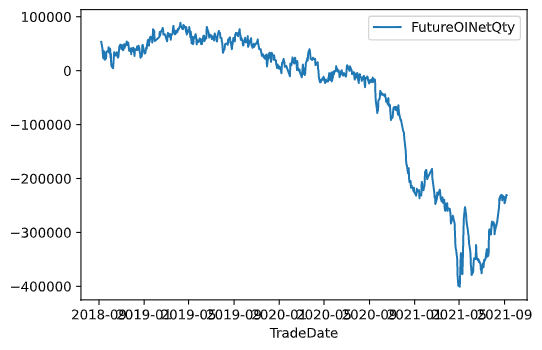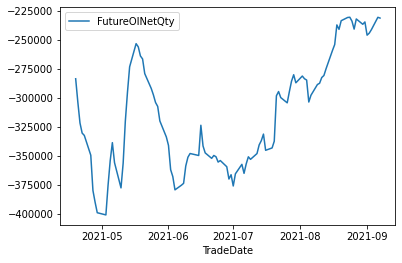# 制作图表

## 改造VS Code

``````pipenv install jupyter
``````## 执行程序

``````matplotlib is required for plotting when the default backend "matplotlib" is selected.
``````

``````import db_connect
import pandas
``````

``````my_connt_obj = db_connect.mysql_connect()
# print(my_connt_obj.db_settings) #for check data
conn = my_connt_obj.connect()
``````

``````df = pandas.read_sql("SELECT TradeDate,FutureOINetQty FROM LegalDailyFutureOption WHERE TradeGroup ='外资及陆资'", con=conn)
``````

``````total_data = df.set_index("TradeDate")
ax1 = total_data.plot()
````````````total_data2 = total_data.iloc[-100:]